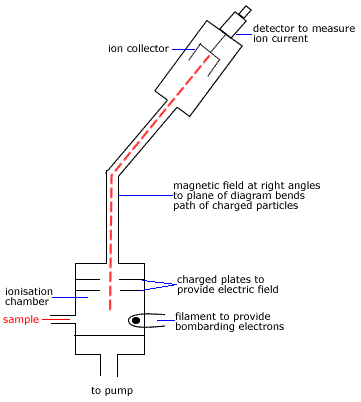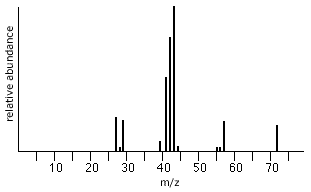# Mass Spectroscopy for Structural Determination Chemistry Tutorial

## Key Concepts

• In a Mass Spectrometer, a molecule is bombarded with a high-energy electron beam causing it to fragment into smaller ions.
• Each of these fragments has its own molecular mass.
• Mass Spectrometers use the difference in mass-to-charge ratio (m/z or m/e) of ionised atoms and molecules to separate them from each other.
• A mass spectrum is the record of the quantity of ions of particular mass-to-charge ratio.
The molecular ion peak is due to ions of the parent molecule and gives us the molecular mass of the molecule.
• Peak heights on a mass spectrum are proportional to the number of ions of each mass.
The most intense peak in the spectrum is called the base peak.
• Mass Spectroscopy can be used to determine:

(a) relative atomic mass of an element

(b) molecular mass of a molecule

(c) structure of a molecule

No ads = no money for us = no free stuff for you!

## The Mass SpectrometerStep 1 sample is vaporised before entering mass spectrometer. M(s) → M(g) or M(l) → M(g) Sample enters the low pressure ionisation chamber where an electron beam ionises the sample. M(g) + e- → M+(g) + 2e- (or   M(g) → M+(g) + e-) Ions are accelerated to high speeds by an electric field. Ions pass through a perpendicular magnetic field which bends the path of the ions dependent on their mass-to-charge ratio. Ionised fragments of the molecule with the greatest mass are deflected the least. Ions with a particular mass reach the collector. Different masses can be collected by changing the magnetic or electric field. The detector identifies the mass of each ion from its path. Data is recorded as a mass spectrum.

Do you know this?

Play the game now!

## Determing Molecular Mass of a Molecule

The simplest event that occurs in the Mass Spectrometer is the removal a single electron from the molecule in the gas phase by the electron beam.

This ion is known as the molecular ion.

The molecular ion is given the symbol(1) M+

 vaporised molecule enters electron beam producing ionised molecule and an electron molecule(g) + e- → molecule+(g) + 2e- M(g) + e- → M+(g) + 2e-

The molecular ion, M+, will have the greatest mass of all the ionised fragments of the molecule so it will be deflected the least by the magnetic field in the Mass Spectrometer.

If some of the molecular ions don't fragment before reaching the detector, they will be recorded as the highest molecular mass (m/z or m/e) peak in the mass spectrum.(2)

For example, methanol (CH3OH) forms the molecular ion(3) CH3OH+

 vaporised methanol enters electron beam producing molecular ion(ionised methanol) and an electron CH3OH(g) + e- → CH3OH+(g) + 2e-

Molecular mass(4) of CH3OH+(g) ≈ 12 + (3 × 1) + 16 + 1 = 32
Charge on the CH3OH+(g) is 1+
mass-to-charge ratio (m/z or m/e) = 32/1 = 32
Mass spectrum of methanol should show a molecular ion peak for methanol(5) at 32

You should note that there may be peaks of greater m/z (m/e) than the molecular ion peak but these peaks are of much lower intensity.
These low intensity peaks are the result of heavier isotopes of the common isotope replacing atoms in the molecule.
For example, deuterium, 2H, has an isotopic mass of 2 while the common isotope of hydrogen, 1H, has an isotopic mass of 1.
If one 1H is replaced by a 2H in a molecule, the molecular mass has increased by 1 but there will be such a small number of these that the intensity of the peak at this m/z (m/e) will be very low.

Do you understand this?

Take the test now!

## Determining Molecular Structure of Molecule

When a molecule is bombarded with the high-energy electron beam it fragments into smaller ions which are then accelerated through the magnetic field before being collected and detected.

In the discussion above we saw that when methanol, CH3OH, enters the electron beam it is ionised by losing an electron and producing the molecular ion CH3OH+

Many of these molecular ions will disintegrate quickly, producing a positively charged fragment of the molecule and a radical

 molecular ion disintegrates producing positively charged fragment and a radical CH3OH+ → CH2OH+ and H. CH3OH+ → CH3+ and OH.

Even the positively charged fragments will disintegrate!
For example, CH2OH+ → CHO+ + H2

Positively charged fragments can be identified in the mass spectrum by their mass-to-charge (m/z or m/e) ratios(6).
The molecular mass of some molecule fragments commonly produced in a mass spectrometer are shown in the table on the right.

Common Mass Spectrum Fragments
m Fragment
14 CH2
15 .CH3
17 .OH
26 .CN
28 H2C=CH2
29 .CH2CH3     .CHO
31 .OCH3
35 .Cl
43 CH3C.=O
45 .OCH2CH3

## Base Peak

Each ion that reaches the detector is recorded. The intensity of a peak in the recording at a particular m/z (m/e) is therefore related to the number of fragments with that mass-to-charge ratio.
A high peak in the mass spectrum indicates that lots of that fragment were produced, a low peak indicates few of those fragments were produced.

The most intense peak (the highest peak) on the mass spectrum is called the base peak.
The base peak is assigned a value of 100%.
The intensities (height × sensitivity factor) of all the other peaks in the spectrum, including the molecular ion peak (if it is not itself the base peak) are reported as percentages of the base peak.

A table of values for m/z (m/e) and corresponding % of base peak values may be presented, but, most likely these values will be presented in the form of a graph.

Can you apply this?

Join AUS-e-TUTE!

Take the exam now!

## Worked Example

Consider pentane, C5H12.

Mass Spectrum of Pentanem Fragment detected loss of
72 CH3CH2CH2CH2CH3+
57 .CH2CH2CH2CH3+ CH3 (m-15)
43 .CH2CH2CH3+ CH2 (m-14)
29 .CH2CH3+ CH2 (m-14)

The highest m/z peak at 72 corresponds to the molecular mass of the pentane, CH3CH2CH2CH2CH3, molecule.

molecular mass (C5H12) = (5 × 12) + (12 × 1) = 60 + 12 = 72

The peak at 57 represents the loss of a CH3 (m=15) fragment from pentane, ie 72 - 15 = 57.

57 is the molecular mass of the CH2CH2CH2CH3+ remaining fragment.

The peak at 43 represents the loss of a CH2 fragment (m=14), ie, 57 - 14 = 43.

43 is the molecular mass of the CH2CH2CH3+ remaining fragment.

The peak at 29 also represents the loss of a CH2 fragment (m=14), ie, 43 - 14 = 29.

29 is the molecular mass of the CH2CH3+ remaining fragment.

The most intense peak, the base peak, at 43 represents the most common fragment detected in the mass spectrometer, CH2CH2CH3+.

Can you apply this?

Join AUS-e-TUTE!

Take the exam now!

Footnotes:

(1) The molecular ion is in fact a radical cation so a better symbol is M.+ in which the single dot represents the odd electron.

(2) Some molecules, such as some alcohols, do not display molecular ion peaks, instead they have a peak at m-1 corresponding to the loss of one hydrogen atom from the parent alcohol.

(3) The molecular ion for methanol can also be represented as the radical cation CH3OH.+

(4) The mass observed for the molecular ion peak is the sum of the exact masses of the most abundant isotopes of carbon, hydrogen and oxygen which differs from the molecular masses based on the weighted average of all the natural isotopes of an element. For this reason it is common to use unit mass resolution results.

(5) and (6) Small peaks surround a main peak due to the natural isotopic abundances of 13C, 2H, etc.